Barry Wright Astek FS6-120A 6-axis force sensorOverview: Barry Wright Astek FS6-120A 6-axis force sensor, measures 3 axis of force and 3 axis of torque6-axis force sensor (3/23/2015): 6 axis force sensor6-axis force sensor (3/23/2015): Sensor, pneumatic cylinder and rotational compensator6-axis force sensor (3/23/2015): Sensor, pneumatic cylinder and rotational compensator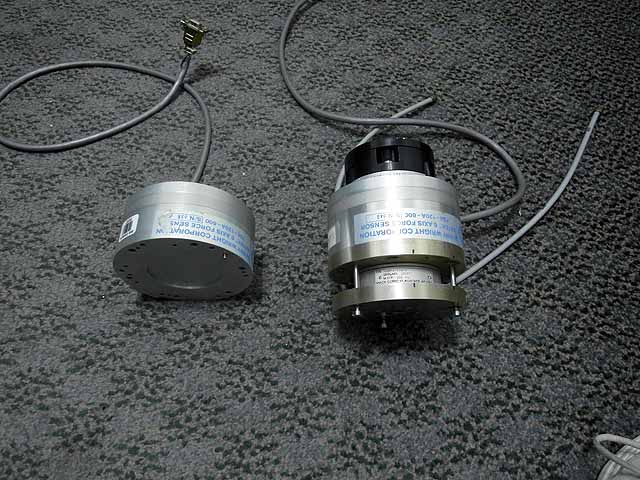6-axis force sensor (3/23/2015): Sensor, pneumatic cylinder and rotational compensator Barry Wright FS6-120A 6-axis force sensor manual Valid commands (15 character max) --------------------------- CF=set frame for n=axis (n=1:3) and x=axis offset (x=-500:+500) CL=set limits for n=axis (n=1:6) and x=limit (x=0:3276 for n=1:3, x=0,327697 for n=4:6) CM= CP=set passband filter for x=(1=240,2=120,3=60,4=30,5=15) CR=set baud rate for x=(1=38400,2=19200,3=9600(default),4=4800,5=2400,6=1200,7=600,8=300) CT=set rate threshold for x=(0 to 4096) DC=display configuration DF=display refference frame DL=display limits DM=display memory for xxxx=address in hex (xxxx=0000 to FFFE) DP=display passband filter DR=display rs232 baud rate DT=display rate threshold EE=enable echo EO=enable data output for x=optional parm (null=ascii,1=ascii,2=16byte,4=16byte) IE=disable echo IL=disable limits IO=disable data output IT=disable rate threshold PC= PP= RB=reset bias RF=reset frame RL=reset limits RT=reset threshold ST=self test DB9 Cable pinout --------------------------- 1=analog output 2=gnd 3=+12v (~320mA) 4=gnd 5=-12v (~190mA) 6=rs232 txd 7=rs232 rxd 8=gnd 9=NC Operating hints --------------------------- EO enables output, in ascii mode it sends back a fixed width 66 character string.6-axis force sensor (3/23/2015): Microprocessor board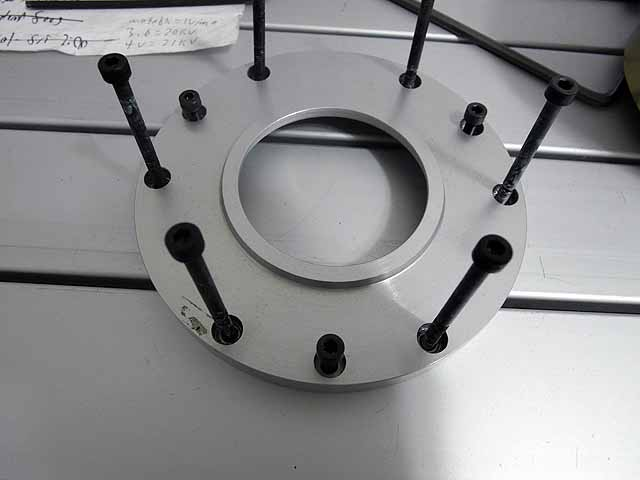6-axis force sensor (3/23/2015): End cap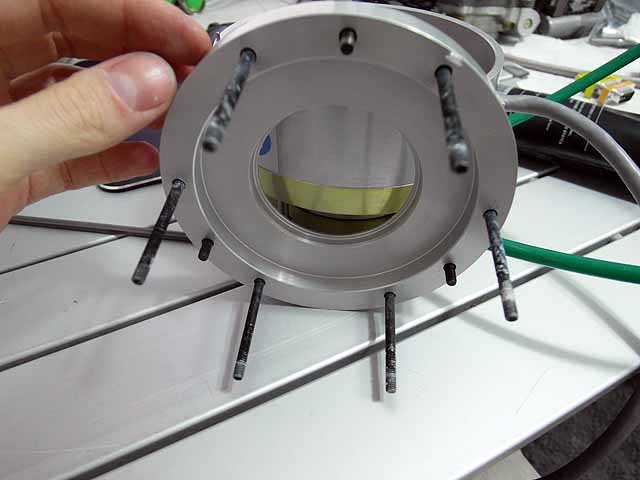6-axis force sensor (3/23/2015): End cap6-axis force sensor (3/23/2015): Mechanical limit plate prevents overstressing transducer.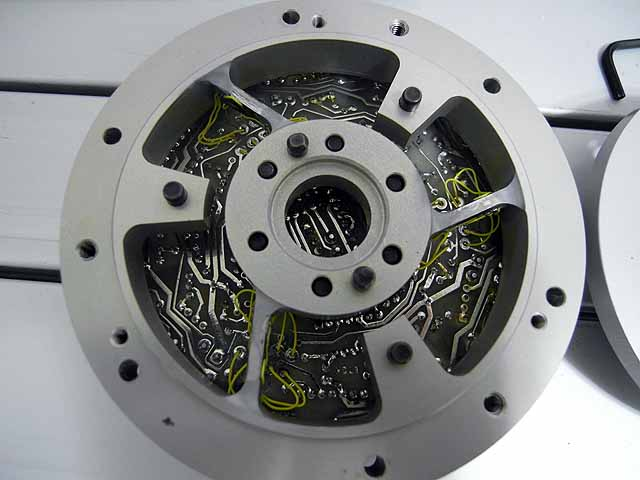6-axis force sensor (3/23/2015): Strain gauges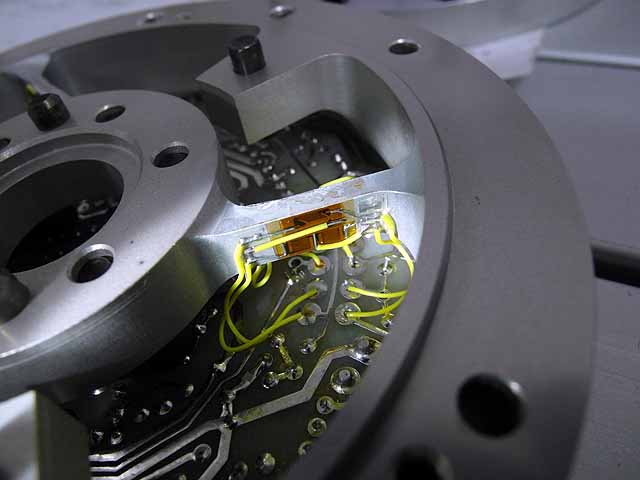6-axis force sensor (3/23/2015): Strain gauges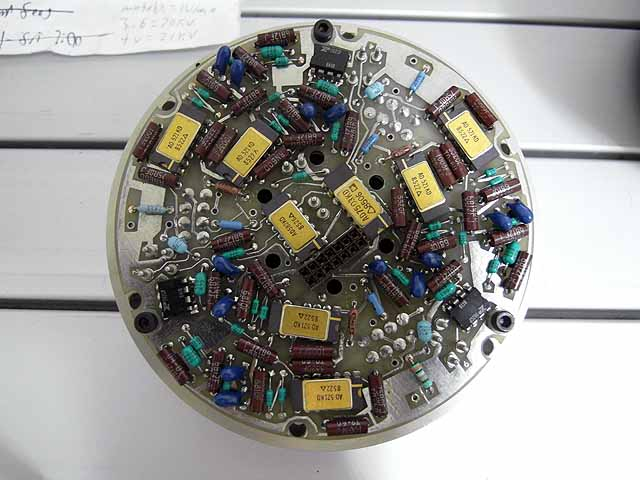6-axis force sensor (3/23/2015): Analog board with precision amplifiers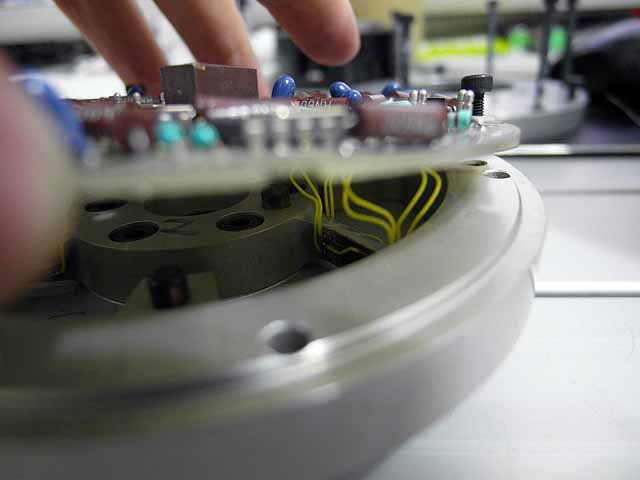6-axis force sensor (3/23/2015): Strain gauges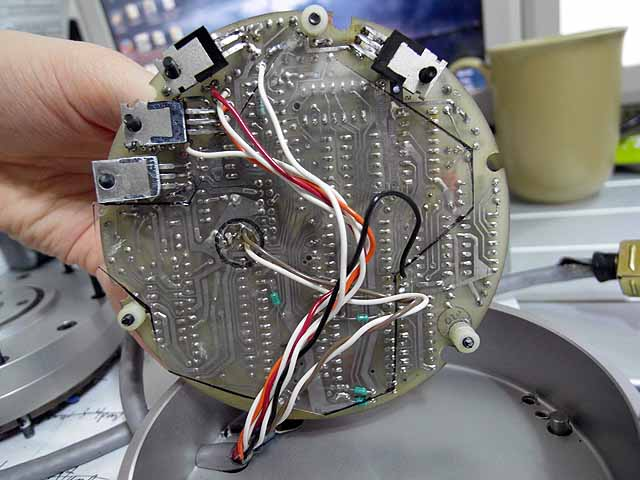6-axis force sensor (3/23/2015): Bottom of microprocessor board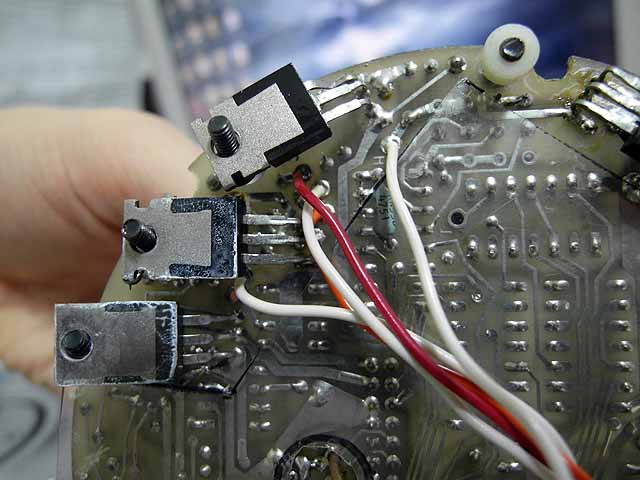6-axis force sensor (3/23/2015): Bottom of microprocessor board6-axis force sensor (3/23/2015): Bottom of microprocessor board6-axis force sensor (3/23/2015): Bottom of microprocessor board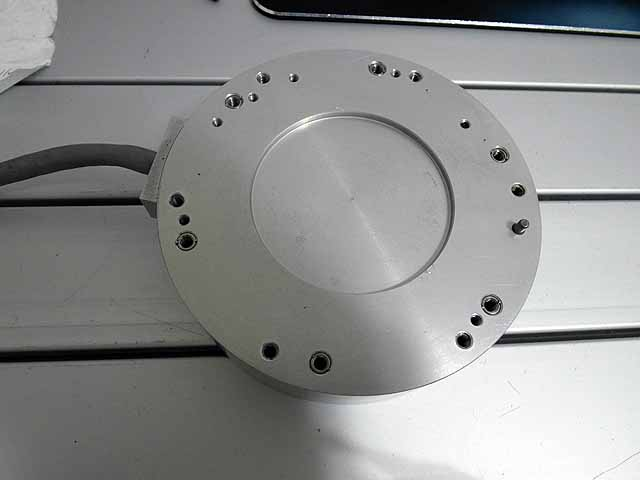6-axis force sensor (3/23/2015): Bottom of force sensor6-axis force sensor (3/23/2015): DC-DC converter to supply +12 and -12v to transducer6-axis force sensor (3/23/2015): DC-DC converter box6-axis force sensor (3/23/2015): DC-DC converter box with components installed6-axis force sensor (3/23/2015): DC-DC converter box with components installed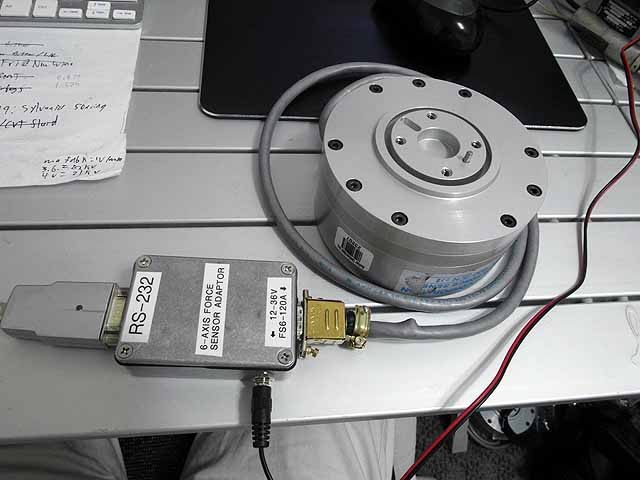6-axis force sensor (3/23/2015): 6-axis sensor connected to RS-232 adapter through DC-DC converter box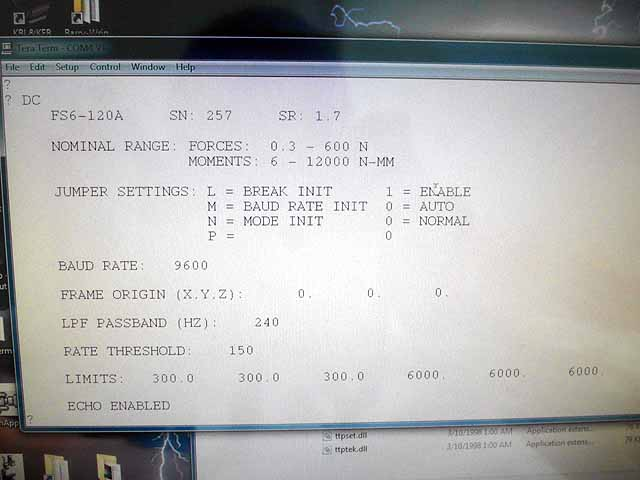6-axis force sensor (3/23/2015): Communicating with censor through terra term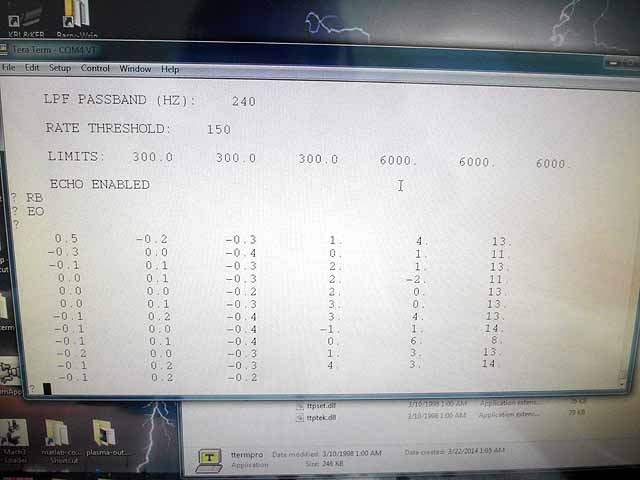6-axis force sensor (3/23/2015): Communicating with censor through terra term, sensor outputs data as 66 character ASCII string containing 6 numbers6-axis force sensor (3/23/2015): Plotting data in MatlabWWW http://www.rtftechnologies.org
 By attempting to reproduce any experiments or devices listed on this domain in part or in whole, you agree to hold me harmless against any lawsuit or liability. Copyright © 1998 - 2005 by Andrew Seltzman. All rights reserved. Contact me at: admin@rtftechnologies.org